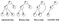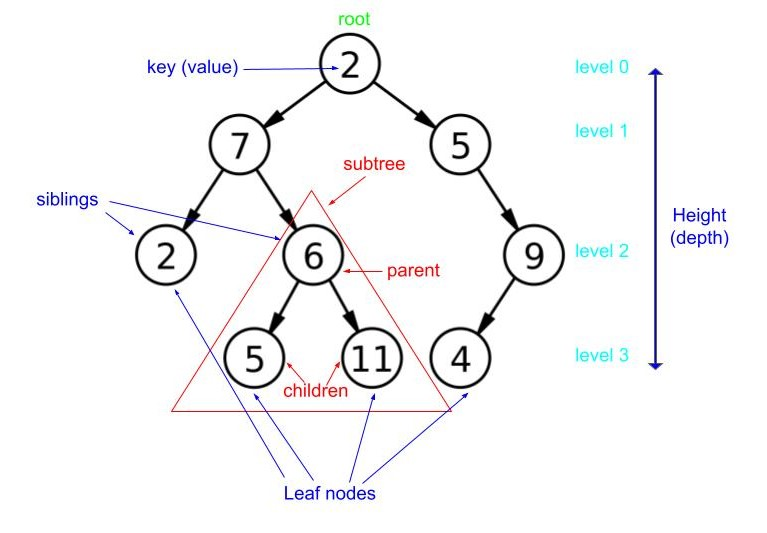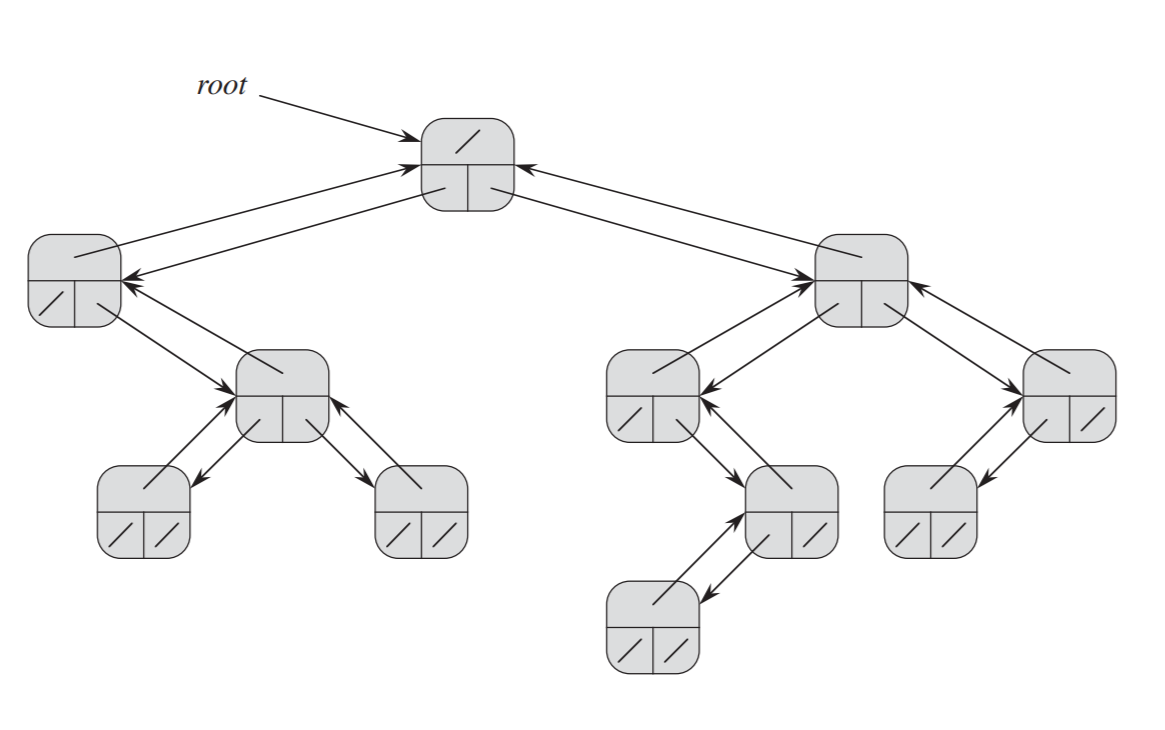# Basic Understanding of Binary Tree

Here we will explore what binary trees are and see how we can implement binary trees in Python. A basic understanding of programming in Python or any programming language is recommended.

First, we need to understand a tree data structure. Unlike Arrays, Linked Lists, Stacks, and Queues, tree represents a hierarchical data structure. The topmost node in a tree is called root and the elements that are under an element are called its children.

Tree Data Structure

• Each node (including the root) has a key (value)
• Each node has only one parent, except the root
• Each node may have zero or more children
• All nodes are reachable from the root

Binary tree is a tree in which each node has a maximum of 2 children; since there are utmost 2 children, we call them left child and right child. The only difference between a tree and a binary tree is the number of children each parent node has. (A tree can have more than 2 children)Figure 1: (image credit: reference 2)

Why Trees?

We use trees when we want to store information that forms a hierarchy, such as storing a file system that has subfolders and files. Also, when it comes to sorting and searching, tress provides a faster way than using Arrays or Linked List.

Properties of Binary Tree

Every node contains following parts:

1. Key (value) of the node
2. Pointer to Parent node
3. Pointer to Left child node
4. Pointer to Right child nodeFigure 2: Binary Tree

In the example binary tree above:

Key (value) of the root: 2

Parent of node 6: node 7

Left child of node 7: node 2

Right child of node 7: node 6

Tree Terminology

Subtree is a smaller tree consisting of all of its descendants inside a tree. In figure 2, the nodes inside the red triangle represent a subtree of the whole binary tree.

Leaf nodes are the nodes that have no children, which means the leaf nodes are always at the bottom of the tree. Nodes 2, 5, 11, 4 are leaf nodes.

Height of the binary tree is the longest path from root node to any leaf node in the tree. The height of binary tree in the example above is 3. The root is at level 0 — meaning that if there is only one node in the tree, the height is 0.

Implementation of a binary tree

Binary Tree can be implemented by representing each node of a tree by an object.Figure 3: Each node x has the attributes x.key (top), x.left (lower left) and x.right (lower right); (image credit: reference 1)

The code block below shows the representation of a node:

`class Node:  def __init__(self, value):    self.key = value     # the key of the node    self.parent = None   # the pointer to the parent node    self.left = None     # the pointer to the left child node    self.right = None    # the pointer to the right child node`

Creating a binary tree shown in Figure 2 above:

`# create a root of a treeroot = Node(2)             # add the topmost node (the root)# We don't have to create parent pointer for the root parent since# the root doesn't have a parent# If you print root.left right after creating the root, you'll see# None because we haven't created any left or right child of the# root yet# create left and right children for the rootroot.left = Node(7)        # the pointer to left childroot.left.parent = root    # the pointer to its parent noderoot.right = Node(5)       # the pointer to right childroot.right.parent = root   # the pointer to its parent node# After creating these nodes, the root is linked to its two children# by left pointer to Node 7, by right pointer to Node 5, and by# parent pointer to root# create left and right children for Node 7 and link to their parentroot.left.left = Node(2)root.left.left.parent = root.left    # parent is node(7)root.left.right = Node(6)root.left.right.parent = root.left# create right child for Node 5root.right.right = Node(9)root.right.right.parent = root.right  # parent is node(5)# At this point, we have created until level 2# create left and right children for Node 6root.left.right.left = Node(5)root.left.right.left.parent = root.left.right  # parent is node(6)root.left.right.right = Node(11)root.left.right.right.parent = root.left.right# create left child for Node 9root.right.right.left = Node(4)root.right.right.left.parent = root.right.right  # parent is node(9)# printing the root and its left and right childrenprint(root.key, root.left.key, root.right.key)`

Feel free to delete some of the nodes and play around until you understand the hierarchical relation of the nodes.

Recursion on Binary Trees

Recursive methods can be used to traverse all the nodes in the binary tree. Let's see how we can print all nodes in the binary tree using recursion.

The naive method to print all the nodes is to go over all the nodes just like we did when we created them. We can also use iteration (loop) to print all the nodes in the tree. However, the iterative approach to print nodes is a bit more complicated; it requires saving the parent nodes and mapping to their children so we will not cover it in this tutorial. Once you are more comfortable with the tree data structure, feel free to try implementing the iterative version.

The code shown below uses recursion to print all the nodes in the tree.

`def print_tree(root):  if root == None:    return  print(root.key)  print_tree(root.left)  print_tree(root.right)`

The code has base case and recursive case:

Base case: check if the current node is None — which means there is no node saved. Cases are when the tree is empty or the traversal reaches the leaf node; then we will return and stop the recursion.

Recursive case: recursively traverse left and right subtrees; to do this we need to call the function itself with the input left child and right child respectively.

`# call print_tree and input root Node>>> print_tree(root)# Output should be like this>>> 2 7 2 6 5 11 5 9 4`

The print_tree function prints the nodes in depth-first order, which means it traverses the left subtrees first then it comes back to print the right subtrees. Of course, this is one example of tree traversal and there are other ways of traversing trees.

I hope this tutorial gives you some knowledge of binary tree data structures and how to represent them. In the next tutorial, we will talk about the full binary tree or heap data structures, and heapsort. See you until then. Chao!

Reference

1. Introduction to Algorithms, Third Edition, Thomas H. Cormen, Charles E. Leiserson, Ronald Rivest, Clifford Stein.
2. https://www.cs.cornell.edu/courses/cs2110/2014fa/L10-Trees/cs2110fa14Trees.pdf

Graduate Teaching Assistant at CSE, University of Minnesota. Love to code and share knowledge with others.

## More from Myat Mo

Graduate Teaching Assistant at CSE, University of Minnesota. Love to code and share knowledge with others.